# Fall 2021 Midterm Exam

Instructor(s): Suraj Rampure, Janine Tiefenbruck

This exam was administered remotely via Gradescope. The exam was open-notes, and students could access any resource including the internet and Jupyter notebooks. Students had 50 minutes to work on it.

King Triton, UCSD’s mascot, is quite the traveler! Throughout this exam, we will be working with the flights DataFrame, which details several facts about each of the flights that King Triton has been on over the past few years. The first few rows of flights are shown below.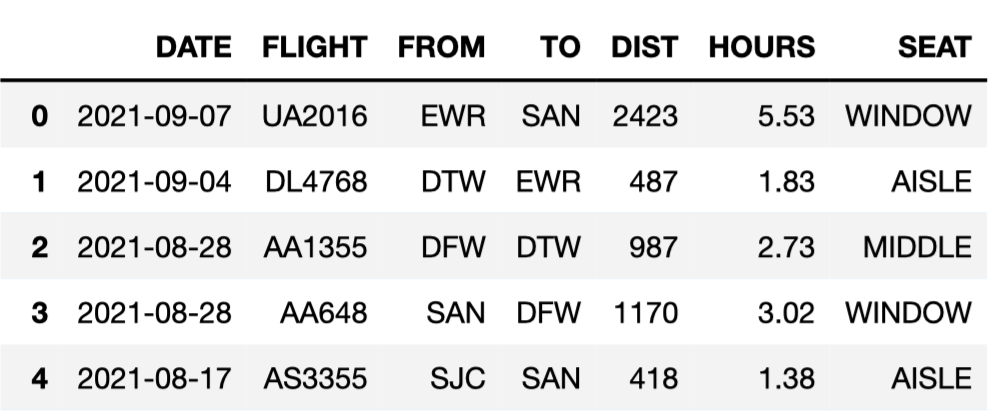Here’s a description of the columns in flights:

• 'DATE': the date on which the flight occurred. Assume that there were no “redeye” flights that spanned multiple days.
• 'FLIGHT': the flight number. Note that this is not unique; airlines reuse flight numbers on a daily basis.
• 'FROM' and 'TO': the 3-letter airport code for the departure and arrival airports, respectively. Note that it’s not possible to have a flight from and to the same airport.
• 'DIST': the distance of the flight, in miles.
• 'HOURS': the length of the flight, in hours.
• 'SEAT': the kind of seat King Triton sat in on the flight; the only possible values are 'WINDOW', 'MIDDLE', and 'AISLE'.

Tip: Open this page in another tab, so that it is easy to refer to this data description as you work through the exam.

## Problem 1

### Problem 1.1

Which of these would it make sense to use as the index of flights?

• 'DATE'

• 'FLIGHT'

• 'FROM'

• 'TO'

• None of these are good choices for the index

Answer: None of these are good choices for the index

When choosing an index, we have to make sure that the index is different for each row of the DataFrame. The index in this case should uniquely identify the flight.

'DATE'does not uniquely identify a flight because there are many different flights in a single day. 'FLIGHT' does not uniquely identify a flight because airlines reuse flight numbers on a daily basis, as we are told in the data description. Neither 'FROM' nor 'TO' uniquely identifies a flight, as there are many flights each day that depart from each airport and arrive at each airport.

Therefore, there is no single column that’s sufficient to uniquely identify a flight, but if we could use multiple columns to create what’s called a multi-index, we’d probably want to use 'DATE' and 'FLIGHT' because each row of our DataFrame should have a unique pair of values in these columns. That’s because airlines don’t reuse flight numbers within a single day.

##### Difficulty: ⭐️⭐️⭐️

The average score on this problem was 57%.

### Problem 1.2

What type of variable is 'FLIGHT'?

• Categorical

• Numerical

'FLIGHT' is a categorical variable because it doesn’t make sense to do arithmetic with the values in the 'FLIGHT' column. 'FLIGHT' is just a label for each flight, and the fact that it includes some numbers does not make it numerical. We could have just as well used letter codes to distinguish flights.

##### Difficulty: ⭐️

The average score on this problem was 98%.

## Problem 2

### Problem 2.1

Which of these correctly evaluates to the number of flights King Triton took to San Diego (airport code 'SAN')?

• flights.loc['SAN'].shape

• flights[flights.get('TO') == 'SAN'].shape

• flights[flights.get('TO') == 'SAN'].shape

• len(flights.sort_values('TO', ascending=False).loc['SAN'])

Answer: flights[flights.get('TO') == 'SAN'].shape

The strategy is to create a DataFrame with only the flights that went to San Diego, then count the number of rows. The first step is to query with the condition flights.get('TO') == 'SAN' and the second step is to extract the number of rows with .shape.

Some of the other answer choices use .loc['SAN'] but .loc only works with the index, and flights does not have airport codes in its index.

##### Difficulty: ⭐️

The average score on this problem was 95%.

### Problem 2.2

Fill in the blanks below so that the result also evaluates to the number of flights King Triton took to San Diego (airport code 'SAN').

flights.groupby(__(a)__).count().get('FLIGHT').__(b)__            

What goes in blank (a)?

• 'DATE'

• 'FLIGHT'

• 'FROM'

• 'TO'

What goes in blank (b)?

• .index

• .index[-1]

• .loc['SAN']

• .iloc['SAN']

• .iloc

True or False: If we change .get('FLIGHT') to .get('SEAT'), the results of the above code block will not change. (You may assume you answered the previous two subparts correctly.)

• True

• False

Answer: 'TO', .loc['SAN'], True

The strategy here is to group all of King Triton’s flights according to where they landed, and count up the number that landed in San Diego. The expression flights.groupby('TO').count() evaluates to a DataFrame indexed by arrival airport where, for any arrival airport, each column has a count of the number of King Triton’s flights that landed at that airport. To get the count for San Diego, we need the entry in any column for the row corresponding to San Diego. The code .get('FLIGHT') says we’ll use the 'FLIGHT' column, but any other column would be equivalent. To access the entry of this column corresponding to San Diego, we have to use .loc because we know the name of the value in the index should be 'SAN', but we don’t know the row number or integer position.

##### Difficulty: ⭐️⭐️

The average score on this problem was 89%.

### Problem 2.3

Consider the DataFrame san, defined below.

san = flights[(flights.get('FROM') == 'SAN') & (flights.get('TO') == 'SAN')]

Which of these DataFrames must have the same number of rows as san?

• flights[(flights.get('FROM') == 'SAN') and (flights.get('TO') == 'SAN')]

• flights[(flights.get('FROM') == 'SAN') | (flights.get('TO') == 'SAN')]

• flights[(flights.get('FROM') == 'LAX') & (flights.get('TO') == 'SAN')]

• flights[(flights.get('FROM') == 'LAX') & (flights.get('TO') == 'LAX')]

Answer: flights[(flights.get('FROM') == 'LAX') & (flights.get('TO') == 'LAX')]

The DataFrame san contains all rows of flights that have a departure airport of 'SAN' and an arrival airport of 'SAN'. But as you may know, and as you’re told in the data description, there are no flights from an airport to itself. So san is actually an empty DataFrame with no rows!

We just need to find which of the other DataFrames would necessarily be empty, and we can see that flights[(flights.get('FROM') == 'LAX') & (flights.get('TO') == 'LAX')] will be empty for the same reason.

Note that none of the other answer choices are correct. The first option uses the Python keyword and instead of the symbol &, which behaves unexpectedly but does not give an empty DataFrame. The second option will be non-empty because it will contain all flights that have San Diego as the departure airport or arrival airport, and we already know from the first few rows of flight that there are some of these. The third option will contain all the flights that King Triton has taken from 'LAX' to 'SAN'. Perhaps he’s never flown this route, or perhaps he has. This DataFrame could be empty, but it’s not necessarily going to be empty, as the question requires.

##### Difficulty: ⭐️⭐️⭐️

The average score on this problem was 70%.

## Problem 3

Fill in the blanks below so that the result is a DataFrame with the same columns as flights plus a new column, 'SPEED', containing the average speed of each flight, in miles per hour.

flights.__(a)__(SPEED=__(b)__)

### Problem 3.1

What goes in blank (a)?

• groupby

• assign

• rename

• drop

• merge

Answer: assign

We want to add a new column, so we must use assign. We can also tell that the answer will be assign because it’s the only DataFrame method that takes an input of the form SPEED=___. Remember that when using assign, we get to call the new column anything we want, and we don’t use quotes around its name.

##### Difficulty: ⭐️

The average score on this problem was 100%.

### Problem 3.2

What goes in blank (b)?

Answer: (flights.get('DIST') / flights.get('HOURS'))

In this blank, we’ll need a Series or array containing the average speed of each flight, in miles per hour.

To calculate the average speed of an individual flight in miles per hour, we’d simply divide the total number of miles by the total amount of time in hours. For example, a flight that travels 500 miles in one hour travels at 500 miles per hour. Note that this is an average speed; at some points of the journey, the plane may have been moving faster than this speed, at other times slower. Because we are calculating an average speed for the whole trip by simply dividing, we don’t need to use .mean().

Once we know how to calculate the average speed for an individual flight, we can do the same operation on each flight all at once using Series arithmetic. flights.get('DIST') is a Series containing the distances of each flight, and flights.get('HOURS') is a Series containing the times of each flight, in the same order. When we divide these two Series, corresponding entries are divided and the result is a Series of average speeds for each flight, as desired.

##### Difficulty: ⭐️

The average score on this problem was 93%.

## Problem 4

We define the seasons as follows:

Season Month
Spring March, April, May
Summer June, July, August
Fall September, October, November
Winter December, January, February

### Problem 4.1

We want to create a function date_to_season that takes in a date as formatted in the 'DATE' column of flights and returns the season corresponding to that date. Which of the following implementations of date_to_season works correctly? Select all that apply.

Option 1:

def date_to_season(date):
month_as_num = int(date.split('-'))
if month_as_num >= 3 and month_as_num < 6:
return 'Spring'
elif month_as_num >= 6 and month_as_num < 9:
return 'Summer'
elif month_as_num >= 9 and month_as_num < 12:
return 'Fall'
else:
return 'Winter'

Option 2:

def date_to_season(date):
month_as_num = int(date.split('-'))
if month_as_num >= 3 and month_as_num < 6:
return 'Spring'
if month_as_num >= 6 and month_as_num < 9:
return 'Summer'
if month_as_num >= 9 and month_as_num < 12:
return 'Fall'
else:
return 'Winter'

Option 3:

def date_to_season(date):
month_as_num = int(date.split('-'))
if month_as_num < 3:
return 'Winter'
elif month_as_num < 6:
return 'Spring'
elif month_as_num < 9:
return 'Summer'
elif month_as_num < 12:
return 'Fall'
else:
return 'Winter' 
• Option 1

• Option 2

• Option 3

• None of these implementations of date_to_season work correctly

Answer: Option 1, Option 2, Option 3

All three options start with the same first line of code: month_as_num = int(date.split('-')). This takes the date, originally a string formatted such as '2021-09-07', separates it into a list of three strings such as ['2021', '09', '07'], extracts the element in position 1 (the middle position), and converts it to an int such as 9. Now we have the month as a number we can work with more easily.

According to the definition of seasons, the months in each season are as follows:

Season Month month_as_num
Spring March, April, May 3, 4, 5
Summer June, July, August 6, 7, 8
Fall September, October, November 9, 10, 11
Winter December, January, February 12, 1, 2

Option 1 correctly assigns months to seasons by checking if the month falls in the appropriate range for 'Spring', then 'Summer', then 'Fall'. Finally, if all of these conditions are false, the else branch will return the correct answer of 'Winter' when month_as_num is 12, 1, or 2.

Option 2 is also correct, and in fact, it does the same exact thing as Option 1 even though it uses if where Option 1 used elif. The purpose of elif is to check a condition only when all previous conditions are false. So if we have an if followed by an elif, the elif condition will only be checked when the if condition is false. If we have two sequential if conditions, typically the second condition will be checked regardless of the outcome of the first condition, which means two if statements can behave differently than an if followed by an elif. In this case, however, since the if statements cause the function to return and therefore stop executing, the only way to get to a certain if condition is when all previous if conditions are false. If any prior if condition was true, the function would have returned already! So this means the three if conditions in Option 2 are equivalent to the if, elif, elif structure of Option 1. Note that the else case in Option 1 is reached when all prior conditions are false, whereas the else in Option 2 is paired only with the if statement immediately preceding it. But since we only ever get to that third if statement when the first two if conditions are false, we still only reach the else branch when all three if conditions are false.

Option 3 works similarly to Option 1, except it separates the months into more categories, first categorizing January and February as 'Winter', then checking for 'Spring', 'Summer', and 'Fall'. The only month that winds up in the else branch is December. We can think of Option 3 as the same as Option 1, except the Winter months have been separated into two groups, and the group containing January and February is extracted and checked first.

##### Difficulty: ⭐️⭐️

The average score on this problem was 76%.

### Problem 4.2

Assuming we’ve defined date_to_season correctly in the previous part, which of the following lines of code correctly computes the season for each flight in flights?

• date_to_season(flights.get('DATE'))

• date_to_season.apply(flights).get('DATE')

• flights.apply(date_to_season).get('DATE')

• flights.get('DATE').apply(date_to_season)

Answer: flights.get('DATE').apply(date_to_season)

Our function date_to_season takes as input a single date and converts it to a season. We cannot input a whole Series of dates, as in the first answer choice. We instead need to apply the function to the whole Series of dates. The correct syntax to do that is to first extract the Series of dates from the DataFrame and then use .apply, passing in the name of the function we wish to apply to each element of the Series. Therefore, the correct answer is flights.get('DATE').apply(date_to_season).

##### Difficulty: ⭐️

The average score on this problem was 97%.

## Problem 5

Suppose we create a DataFrame called socal containing only King Triton’s flights departing from SAN, LAX, or SNA (John Wayne Airport in Orange County). socal has 10 rows; the bar chart below shows how many of these 10 flights departed from each airport.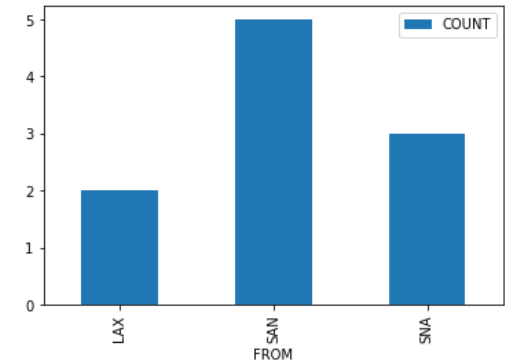Consider the DataFrame that results from merging socal with itself, as follows:

double_merge = socal.merge(socal, left_on='FROM', right_on='FROM')

How many rows does double_merge have?

There are two flights from LAX. When we merge socal with itself on the 'FROM' column, each of these flights gets paired up with each of these flights, for a total of four rows in the output. That is, the first flight from LAX gets paired with both the first and second flights from LAX. Similarly, the second flight from LAX gets paired with both the first and second flights from LAX.

Following this logic, each of the five flights from SAN gets paired with each of the five flights from SAN, for an additional 25 rows in the output. For SNA, there will be 9 rows in the output. The total is therefore 2^2 + 5^2 + 3^2 = 4 + 25 + 9 = 38 rows.

##### Difficulty: ⭐️⭐️⭐️⭐️⭐️

The average score on this problem was 27%.

## Problem 6

We define a “route” to be a departure and arrival airport pair. For example, all flights from 'SFO' to 'SAN' make up the “SFO to SAN route”. This is different from the “SAN to SFO route”.

Fill in the blanks below so that most_frequent.get('FROM').iloc and most_frequent.get('TO').iloc correspond to the departure and destination airports of the route that King Triton has spent the most time flying on.

most_frequent = flights.groupby(__(a)__).__(b)__
most_frequent = most_frequent.reset_index().sort_values(__(c)__)

### Problem 6.1

What goes in blank (a)?

Answer: ['FROM', 'TO']

We want to organize flights by route. This means we need to group by both 'FROM' and 'TO' so any flights with the same pair of departure and arrival airports get grouped together. To group by multiple columns, we must use a list containing all these column names, as in flights.groupby(['FROM', 'TO']).

##### Difficulty: ⭐️⭐️⭐️

The average score on this problem was 72%.

### Problem 6.2

What goes in blank (b)?

• count()

• mean()

• sum()

• max()

Answer: sum()

Every .groupby command needs an aggregation function! Since we are asked to find the route that King Triton has spent the most time flying on, we want to total the times for all flights on a given route.

Note that .count() would tell us how many flights King Triton has taken on each route. That’s meaningful information, but not what we need to address the question of which route he spent the most time flying on.

##### Difficulty: ⭐️⭐️⭐️

The average score on this problem was 58%.

### Problem 6.3

What goes in blank (c)?

• by='HOURS', ascending=True

• by='HOURS', ascending=False

• by='HOURS', descending=True

• by='DIST', ascending=False

Answer: by='HOURS', ascending=False

We want to know the route that King Triton spent the most time flying on. After we group flights by route, summing flights on the same route, the 'HOURS' column contains the total amount of time spent on each route. We need most_frequent.get('FROM').iloc and most_frequent.get('TO').iloc to correspond with the departure and destination airports of the route that King Triton has spent the most time flying on. To do this, we need to sort in descending order of time, to bring the largest time to the top of the DataFrame. So we must sort by 'HOURS' with ascending=False.

##### Difficulty: ⭐️

The average score on this problem was 94%.

## Problem 7

The seat-back TV on one of King Triton’s more recent flights was very dirty and was full of fingerprints. The fingerprints made an interesting pattern. We’ve stored the x and y positions of each fingerprint in the DataFrame fingerprints, and created the following scatterplot using

fingerprints.plot(kind='scatter', x='x', y='y')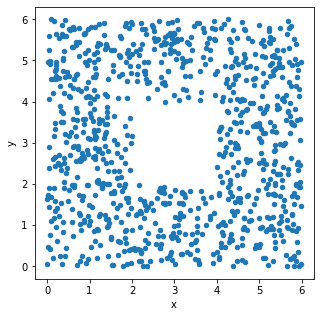### Problem 7.1

True or False: The histograms that result from the following two lines of code will look very similar.

fingerprints.plot(kind='hist',
y='x',
density=True,
bins=np.arange(0, 8, 2))

and

fingerprints.plot(kind='hist',
y='y',
density=True,
bins=np.arange(0, 8, 2))
• True

• False

The only difference between the two code snippets is the data values used. The first creates a histogram of the x-values in fingerprints, and the second creates a histogram of the y-values in fingerprints.

Both histograms use the same bins: bins=np.arange(0, 8, 2). This means the bin endpoints are [0, 2, 4, 6], so there are three distinct bins: [0, 2), [2, 4), and [4, 6]. Remember the right-most bin of a histogram includes both endpoints, whereas others include the left endpoint only.

Let’s look at the x-values first. If we divide the scatterplot into nine equally-sized regions, as shown below, note that eight of the nine regions have a very similar number of data points.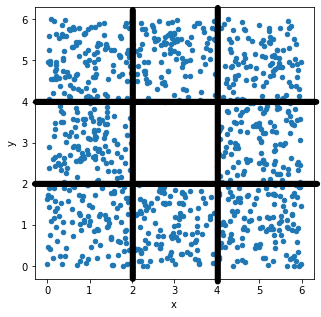Aside from the middle region, about \frac{1}{8} of the data falls in each region. That means \frac{3}{8} of the data has an x-value in the first bin [0, 2), \frac{2}{8} of the data has an x-value in the middle bin [2, 4), and \frac{3}{8} of the data has an x-value in the rightmost bin [4, 6]. This distribution of x-values into bins determines what the histogram will look like.

Now, if we look at the y-values, we’ll find that \frac{3}{8} of the data has a y-value in the first bin [0, 2), \frac{2}{8} of the data has a y-value in the middle bin [2, 4), and \frac{3}{8} of the data has a y-value in the last bin [4, 6]. That’s the same distribution of data into bins as the x-values had, so the histogram of y-values will look just like the histogram of y-values.

Alternatively, an easy way to see this is to use the fact that the scatterplot is symmetric over the line y=x, the line that makes a 45 degree angle with the origin. In other words, interchanging the x and y values doesn’t change the scatterplot noticeably, so the x and y values have very similar distributions, and their histograms will be very similar as a result.

##### Difficulty: ⭐️⭐️

The average score on this problem was 88%.

### Problem 7.2

Below, we’ve drawn a histogram using the line of code

fingerprints.plot(kind='hist',
y='x',
density=True,
bins=np.arange(0, 8, 2))

However, our Jupyter Notebook was corrupted, and so the resulting histogram doesn’t quite look right. While the height of the first bar is correct, the histogram doesn’t contain the second or third bars, and the y-axis is replaced with letters.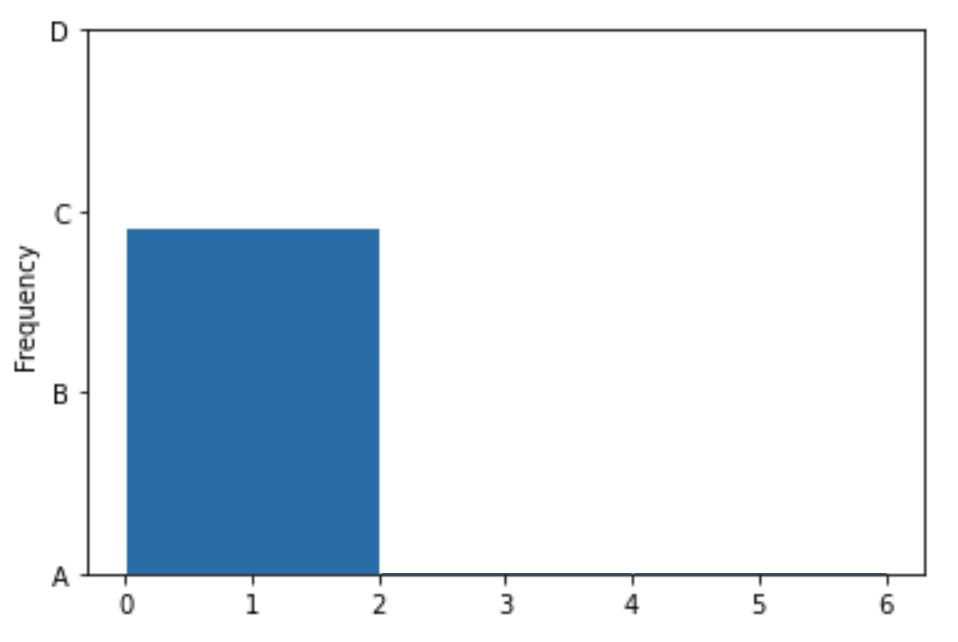Which of the four options on the y-axis is closest to where the height of the middle bar should be?

• A

• B

• C

• D

Which of the four options on the y-axis is closest to where the height of the rightmost bar should be?

• A

• B

• C

• D

We’ve already determined that the first bin should contain \frac{3}{8} of the values, the middle bin should contain \frac{2}{8} of the values, and the rightmost bin should contain \frac{3}{8} of the values. The middle bar of the histogram should therefore be two-thirds as tall as the first bin, and the rightmost bin should be equally as tall as the first bin. The only reasonable height for the middle bin is B, as it’s closest to two-thirds of the height of the first bar. Similarly, the rightmost bar must be at height C, as it’s the only one close to the height of the first bar.

##### Difficulty: ⭐️

The average score on this problem was 94%.

## Problem 8

It turns out that King Triton is so busy that he doesn’t even book his own flights – he has a travel agent who books his flights for him. He doesn’t get to choose the airline that he flies on, but his travel agent gave him the following table, which describes the probability of each of his flights in 2022 being on Delta, United, American, or another airline:

Airline Chance
Delta 0.4
United 0.3
American 0.2
All other airlines 0.1

The airline for one flight has no impact on the airline for another flight.

For this question, suppose that King Triton schedules 3 flights for January 2022.

### Problem 8.1

What is the probability that all 3 flights are on United? Give your answer as an exact decimal between 0 and 1 (not a Python expression).

For all three flights to be on United, we need the first flight to be on United, and the second, and the third. Since these are independent events that do not impact one another, and we need all three flights to separately be on United, we need to multiply these probabilities, giving an answer of 0.3*0.3*0.3 = 0.027.

Note that on an exam without calculator access, you could leave your answer as (0.3)^3.

##### Difficulty: ⭐️

The average score on this problem was 93%.

### Problem 8.2

What is the probability that all 3 flights are on Delta, or all on United, or all on American? Give your answer as an exact decimal between 0 and 1 (not a Python expression).

We already calculated the probability of all three flights being on United as (0.3)^3 = 0.027. Similarly, the probability of all three flights being on Delta is (0.4)^3 = 0.064, and the probability of all three flights being on American is (0.2)^3 = 0.008. Since we cannot satisfy more than one of these conditions at the same time, we can separately add their probabilities to find a total probability of 0.027 + 0.064 + 0.008 = 0.099.

##### Difficulty: ⭐️⭐️

The average score on this problem was 76%.

### Problem 8.3

True or False: The probability that all 3 flights are on the same airline is equal to the probability you computed in the previous subpart.

• True

• False

It’s not quite the same because the previous subpart doesn’t include the probability that all three flights are on the same airline which is not one of Delta, United, or American. For example, there is a small probability that all three flights are on Allegiant or all three flights are on Southwest.

##### Difficulty: ⭐️

The average score on this problem was 90%.

## Problem 9

King Triton has boarded a Southwest flight. For in-flight refreshments, Southwest serves four types of cookies – chocolate chip, gingerbread, oatmeal, and peanut butter.

The flight attendant comes to King Triton with a box containing 10 cookies:

• 4 chocolate chip
• 2 oatmeal, and
• 1 peanut butter

The flight attendant tells King Triton to grab 2 cookies out of the box without looking.

Fill in the blanks below to implement a simulation that estimates the probability that both of King Triton’s selected cookies are the same.

# 'cho' stands for chocolate chip, 'gin' stands for gingerbread,
# 'oat' stands for oatmeal, and 'pea' stands for peanut butter.

cookie_box = np.array(['cho', 'cho', 'cho', 'cho', 'gin',
'gin', 'gin', 'oat', 'oat', 'pea'])

repetitions = 10000
prob_both_same = 0
for i in np.arange(repetitions):
grab = np.random.choice(__(a)__)
if __(b)__:
prob_both_same = prob_both_same + 1
prob_both_same = __(c)__

### Problem 9.1

What goes in blank (a)?

• cookie_box, repetitions, replace=False

• cookie_box, 2, replace=True

• cookie_box, 2, replace=False

• cookie_box, 2

Answer: cookie_box, 2, replace=False

We are told that King Triton grabs two cookies out of the box without looking. Since this is a random choice, we use the function np.random.choice to simulate this. The first input to this function is a sequence of values to choose from. We already have an array of values to choose from in the variable cookie_box. Calling np.random.choice(cookie_box) would select one cookie from the cookie box, but we want to select two, so we use an optional second parameter to specify the number of items to randomly select. Finally, we should consider whether we want to select with or without replacement. Since cookie_box contains individual cookies and King Triton is selecting two of them, he cannot choose the same exact cookie twice. This means we should sample without replacement, by specifying replace=False. Note that omitting the replace parameter would use the default option of sampling with replacement.

##### Difficulty: ⭐️

The average score on this problem was 92%.

### Problem 9.2

What goes in blank (b)?

Answer: grab == grab

The idea of a simulation is to do some random process many times. We can use the results to approximate a probability by counting up the number of times some event occurred, and dividing that by the number of times we did the random process. Here, the random process is selecting two cookies from the cookie box, and we are doing this 10,000 times. The approximate probability will be the number of times in which both cookies are the same divided by 10,000. So we need to count up the number of times that both randomly selected cookies are the same. We do this by having an accumulator variable that starts out at 0 and gets incremented, or increased by 1, every time both cookies are the same. The code has such a variable, called prob_both_same, that is initialized to 0 and gets incremented when some condition is met.

We need to fill in the condition, which is that both randomly selected cookies are the same. We’ve already randomly selected the cookies and stored the results in grab, which is an array of length 2 that comes from the output of a call to np.random.choice. To check if both elements of the grab array are the same, we access the individual elements using brackets with the position number, and compare using the == symbol to check equality. Note that at the end of the for loop, the variable prob_both_same will contain a count of the number of trials out of 10,000 in which both of King Triton’s cookies were the same flavor.

##### Difficulty: ⭐️⭐️

The average score on this problem was 79%.

### Problem 9.3

What goes in blank (c)?

• prob_both_same / repetitions

• prob_both_same / 2

• np.mean(prob_both_same)

• prob_both_same.mean()

Answer: prob_both_same / repetitions

After the for loop, prob_both_same contains the number of trials out of 10,000 in which both of King Triton’s cookies were the same flavor. We’d like it to represent the approximate probability of both cookies being the same flavor, so we need to divide the current value by the total number of trials, 10,000. Since this value is stored in the variable repetitions, we can divide prob_both_same by repetitions.

##### Difficulty: ⭐️

The average score on this problem was 93%.

## Problem 10

Note: This problem is out of scope; it covers material no longer included in the course.

In response to the pandemic, some airlines chose to leave middle seats empty, while others continued seating passengers in middle seats. Let’s suppose Delta did not seat passengers in middle seats during the pandemic, and United did seat passengers in middle seats during the pandemic.

Delta wants to know whether customers were satisfied with them for making this decision not to use middle seats. Suppose they have access to a dataset of customer satisfaction surveys, taken annually for each airline. How can Delta determine whether its new seating policy caused an increase in customer satisfaction?

• Compare Delta’s average customer satisfaction before and after this change went into effect.

• Compare Delta’s average customer satisfaction after the change went into effect to United’s average customer satisfaction at the same point in time.

• Compare the change in Delta’s average customer satisfaction to the change in United’s average customer satisfaction, throughout the same period of time, spanning the change.

• None of the above.